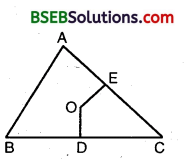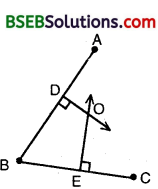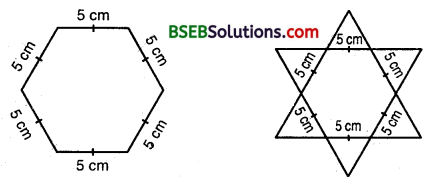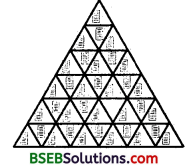# Bihar Board Class 9th Maths Solutions Chapter 7 Triangles Ex 7.5

Bihar Board Class 9th Maths Solutions Chapter 7 Triangles Ex 7.5 Textbook Questions and Answers.

## BSEB Bihar Board Class 9th Maths Solutions Chapter 7 Triangles Ex 7.5Question 1.
ABC is A triangle. Locate a point in the interior of ∆ ABC which is equidistant from all the vertices of ∆ ABC.
Solution:
Let OD and OE be the A perpendicular bisectors of sides BC arid CA of ∆ ABC.∴ O is equidistant from two ends B and C of line-segment BC as O lies on the perpendicular bisector of BC.
Similarly, O is equidistant from C and A.
Thus, the point , of intersection O of the perpendicular bisectors of sides BC, CA and AB is the required point.Question 2.
In a triangle locate a point in its interior which is equidistant from all the sides of the triangle.
Solution:
Let BE and CF be the bisectors of ∠ABC and ∠ACB respectively intersecting AC and AB at E and F respectively.Since. O lies on BE, the bisector of ∠ABC, hence O will be equidistant from AB and BC. Again, O lies on the bisector CF of ∠ACB. Hence, O will be equidistant from BC and AC. Thus, O will be equidistant from AB, BC and CA.Question 3.
In a huge park, people are concentrated at three points (see figure):
A : where there are different slides and swings for children,
B : near which a man-made iake is situated,
C : which is near to a large parking and exit.
Where should an icecream parlour be set up so that maximum number of persons can approach it?
Solution:
The parlour should be equidistant from A, B and C, for which the point of intersection of perpendicular bisector should be located.Thus, O is the required point which is equidistant from A, B and C.Question 4.
Complete the hexagonal and star Rangolies [see figure (i) and (ii)] by filling them with as many equilateral triangles of side 1 cm as you can. Count the number of triangles in each case. Which has more triangles ?Solution:
On filling each figure with equilateral triangles of side 1 cm, we find in figure (i) number of such triangles is 1∠50, and in figure (ii) number of such triangle is 300.